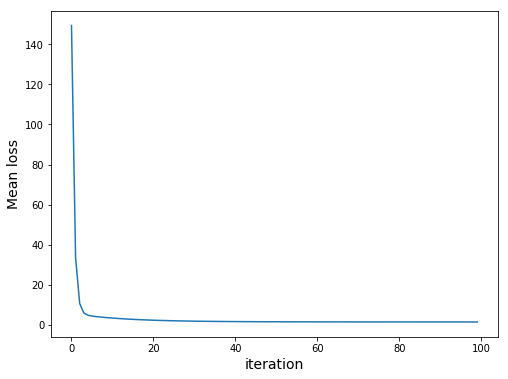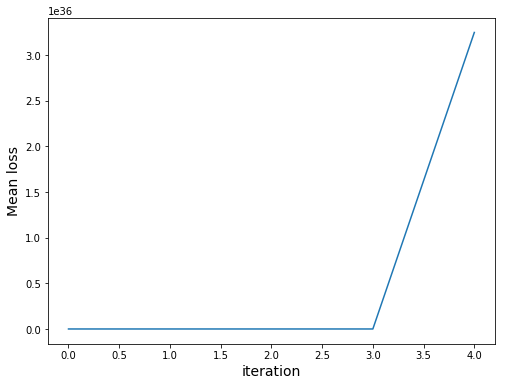# Machine Learning Part 1 — Linear regression in MXNet

This is the first part of the Machine Learning series. For your convenience you can find other parts using the links below (or by guessing the address):
Part 1 — Linear regression in MXNet
Part 2 — Linear regression in SQL
Part 3 — Linear regression in SQL revisited
Part 4 — Linear regression in T-SQL
Part 5 — Linear regression
Part 6 — Matrix multiplication in SQL
Part 7 — Forward propagation in neural net in SQL
Part 8 — Backpropagation in neural net in SQL

In this series I assume you do know basics of machine learning. I will provide some source code for different use cases but no extensive explanation. Let’s go.

Today we will take a look at linear regression in MXNet. We will predict sepal length in well know iris dataset.

We can see some records with `print df.head(3)` or check different iris categories with `df.iris.unique()`.

We have one target variable and four features. Let’s create two adttional:

Two features similar to one hot encoding of categorical feature.

Time to prepare training and test datasets with: `df_train, df_test = train_test_split( df, test_size=0.3, random_state=1)`

Let’s get down to training. We start with defining the training variables and the target one:

Let’s prepare class representing data instance:

We initialize parameters and attach gradient calculation. This is a very nice feature, we don’t need to take care of derivatives, everything is taken care for us.

Let’s now carry on with a single step for gradient:

We just subtract gradient multiplied by learning rate. Also, we use `x[:]` instead of `x` to avoid reinitializing the gradient. If we go with the latter, we will see the following error:

Now, let’s train our model:

We should get the following:Note that our `loss` uses mean whereas we could calculate just a `sum`. However, due to overflow problems we would get the following:Finally, let’s check the performance of trained model:

Done.

# Summary

We can see that linear regression is pretty concise and easy. However, this uses Python and Spark which me might want to avoid. In next parts we will take a look at different solutions.